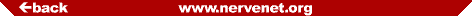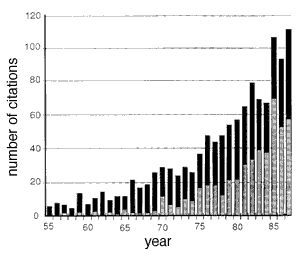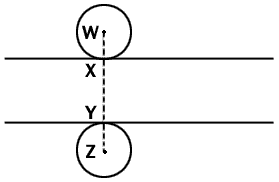## The Annotated Abercrombie

Originally published in The Anatomical Record, Volume 94, pp. 239-247 (1946) by the Wistar Institute of Anatomy and Biology, Philadelphia, PA. Annotations by Robert W. Williams (1998). Last update by RW, 27 November, 2001.

Directory and OutlineCitation frequency of Abercrombie's 1946 paper in neuroscience journals (gray bars) and in all other journals (black bars). These data were extracted from the Science Citation Index, Institute of Scientific Information, Inc., 1955–1987. Roughly 500 of the 1,205 citations listed in the index were published in The Journal of Comparative Neurology, the largest number for any journal. The incidence of miscitation of Abercrombie's paper is high. For example, the first page number is given incorrectly by more than 133 (11%) of the papers. This figure is from Williams and Rakic, 1988.

NOTE TO THE READER: Brackets [...] are used to denote annotations by R. Williams. Brackets are also used to indicate page breaks so that reference can be made to the original page numbers of the 1946 publication.

[page 239 Top]

### ESTIMATION OF NUCLEAR POPULATION FROM MICROTOME SECTIONS

#### M. ABERCROMBIE

Department of Zoology, The University, Birmingham, England

###### ONE FIGURE

Estimations of the numbers of nuclei in microtome sections are frequently made in some branches of histology, particularly in the study of endocrine glands, hemopoietic organs and the central nervous system. The curious neglect of this and indeed of all quantitative methods in most other fields of histology will no doubt soon be a thing of the past. It is therefore important to consider how best to get reliable conclusions from such nuclear counts. The present note deals with a commonly neglected difficulty which necessarily follows from the use of microtome sections. Although I confine discussion to cell nuclei, the argument applies of course to any discrete component of tissue.

The number of nuclei visible in a microtome section 1 can easily be counted. But not all of the objects thus counted are whole nuclei. Some must be fragments of nuclei, because some nuclei lie partly within the section examined, partly within an adjacent section. It is impossible always to tell which are fragmentary, which whole. One cannot therefore extrapolate from the mean apparent number of nuclei within the volume of a microtome section to the mean number within a cubic millimeter or a given mass of tissue without exaggeration; since the count on a single section represents the [page 240] nuclear content of the neighboring tissue which contains the other portions of the transected nuclei. Only if the average dimension of the nuclei in the direction at right angles to that of the section is minute in relation to the thickness of the section, will the exaggeration be negligible. The bigger this nuclear dimension is in relation to section thickness, the bigger the error, because the higher the proportion of nuclear fragments. Agduhr (’41) clearly recognized this source of error.

Even if we work in an entirely relative way, and attempt to compare the nuclear populations of different tissues, or of the same tissue under different conditions, we may be led into error. For obviously any difference in size of the nuclei in the tissues compared will by itself lead to different counts.

We can put these considerations more exactly as follows. The true density of population, from which can be derived by simple proportion the number of nuclei in any volume of tissue, is for our purposes best regarded as the number of nuclear-points within unit volume. By a nuclear-point I mean any geometrical point of the same relative position in all nuclei; say midway along the dimension at right angles to the microtome section. A nuclear-point cannot overlap two adjacent sections, and the number of nuclear-points in a section can therefore be extrapolated to the number in any volume of tissue without error, and can serve for exact comparison of nuclear population in different tissues. If P is the average number of nuclear points per section, A is the crude count of number of nuclei seen in the section, M is the thickness (in µ) of the section, and L the average length (in µ) of the nuclei, then

```         M
P = A ———-               (1)
L + M
```

The derivation of this equation is explained in figure 1 [RW1]. It means that those nuclei whose nuclear-points are outside the section but which are nevertheless represented, by fragments, inside the section, have their nuclear-points extending through [page 241 top] a volume of tissue of (on the average) the thickness of the mean nuclear length. Since microtome sections are commonly roughly the same thickness as the nuclear length, the error in extrapolating from A to the number of nuclei per unit volume of tissue may often reach 100%. The error in comparing on the basis of counts in sections two tissues whose nuclear size is different is much less serious, since it follows from equation (1) that a given change in L produces a disproportionately smaller change in A when P is held constant [RW2].Fig. 1  Diagrammatic side view of a microtome section which lies between the two lines. Two spherical nuclei of equal radius are so place that they touch the surfaces of the sections at X and Y. Any nucleus of this radius will be represented in the section if its center lies anywhere between points W and Z. Since we can assume a random distribution of nuclei between these two points, the proportion of all the nuclei represented in the section whose centers lie within the section is given by XY/WZ, that is section thickness/(section thickness + nuclear diameter). The argument applies statistically to nuclei of any size or shape.

The neglect of these considerations of nuclear size could be illustrated by numerous examples. I have selected a few instances from important papers of recent years. Kindred (’42) records many differential cell counts made on sections of hemopoietic tissues, without consideration of differences in nuclear size, and he apparently extrapolates directly from section populations to populations of whole tissues. Kirkman (’43) in his pioneering study of the cell composition of kidney, compares fibrocyte and macrophage content of different organs, and extrapolates from section counts to the whole organ, apparently regardless of nuclear size. Kirkman [page 242] (’37), in common with many other investigators of the pituitary, in comparing relative numbers of different cell types in the pituitary under different conditions makes no allowance for changes in nuclear size. He records, it is interesting to note, a parallel increase in number and size of basophils, and decrease in number and size of acidophils, after gonadectomy. Clearly it is possible that the size changes account for the numerical changes. Agduhr (’41) has criticised work on the central nervous system, and I will not add any example in this field. Finally, I wonder whether the size of mitotic figures is not sometimes relevant in the very numerous investigations of mitotic rate.

The neglect of nuclear size in these and similar papers may often be harmless, but at least we ought to know that it is harmless. This can only be achieved, and gross errors of extrapolation avoided, if we can obtain the true density of nuclear, that is the density of nuclear-point, populations of tissues. We need therefore a method of obtaining the number of nuclear points in a microtome section. I here suggest two methods, both of which I have used, in collaboration with Dr. M. L. Johnson [RW3], in a study of the nuclei of peripheral nerves (J. Anat. Lond. in the press).

### Method 1

If the average nuclear dimension at right angles to the plane of section (hereafter called nuclear length) is known, the number of nuclear-points within the section (P) can be obtained by means of equation (1) [RW4]. Rather surprisingly, a good approximation to the mean nuclear length can be obtained in many tissues by measuring it directly in sections cut at right angles to the plane of the sections counted. This of course means that nuclear length has be measured on a sample of tissue other than that on which the nuclear counts are done, and sufficient material, homogeneous enough, must be available for this [RW5].

The measurements of overall nuclear length are made, by micrometer eye-piece, on a random sample of the apparent nuclei in the section. Clearly the mean nuclear length so obtained must be less than the true mean nuclear length because [page 243] we cannot differentiate whole nuclei from shorter nuclear fragments. The mean length of all parallel chords of a circle or of an ellipse (in the direction of one of the axes) is 79% of the diameter (actually PI/4 X diameter, Dr. J. H. Waterhouse informs me). It is probably an approximation adequate for many tissues to treat nuclei as spheres or oriented ellipsoids, and we might therefore expect a systematic error of about 21% when we measure the mean nuclear length in sections of these tissues. We do not, however, in practice measure this mean length, since the microtome section is of finite thickness, and therefore, the full length of the nuclei is disproportionately frequently included. If for instance we consider spherical nuclei of average diameter 10 µ, whose length is measured in sections 10 µ thick, the theoretical 21% underestimation is halved. Furthermore, this 11% underestimate does not become translated into an equivalent overestimation of the number of nuclear-points per unit volume, as can be seen from equation (1). The error is in fact nearly halved again, so that in our example the final overestimation of density of nuclear-point population is only 6%. The value of L which, in carrying out this method, we insert in equation (1) is really (assuming nuclear shape as described) equivalent to L - (0.21T)/(T+N) L, where L is the true mean nuclear length, N is the thickness of section in which the nuclear lengths are measured, and T is the mean thickness of nuclei (their dimension at right angles to these sections)[RW6]. The thicker the sections used for counts or measuring (the bigger M and N), the smaller the error; the smaller either of the nuclear dimensions concerned (L and T), the smaller the error. The error of overestimation will in practice seldom exceed 10%.

The 10% overestimation of population density is negligible for most purposes, and it contrasts satisfactorily with the overestimation resulting when no correction is made for nuclear size. If we are primarily interested in comparing the density of population of two tissues, we are on safer ground still, since the error in both densities is in the same direction.

[page 244]

This method was used by Linderstrøm-Lang, Holter and Ohlsen (’35); they did not discuss the size of the systematic error in getting nuclear length from sections. Agduhr (’41) also described an analogous method, which had been used by Carlson, Gustafsson and Möller (’37). Agduhr likewise did not discuss the difficulties of obtaining nuclear dimensions. He proposed a formidable formula by which the counts on sections could be reduced to the true nuclear population. Since it may lead to confusion we must briefly discuss his formula which was:

```       2SM - L
P = A —————               (2)
SM (S + 1)
```

This has been translated in the symbols used above, except that S stands for the whole number of sections through or into which the average nucleus extends. This formula is an approximation, since S is always taken as a whole number. If S is made accurately equal to L/M, then the formula simply reduces to equation (1) [RW7]. Agduhr’s formula is therefore unnecessarily complicated, though of course it gives valuable results.

### Method 2

It is apparent from the preceding discussion that the nuclei represented in any section consist of those whose nuclear-points lie within the section (which we will call group A), and those whose nuclear-points lie outside (group B). Group A gives us the true density of population of the section; the size of group B depends on the average nuclear length. If we compare the number of apparent nuclei we can count in each of two consecutive sections of very different thickness, the thicker section will of course contain more than the thinner. This is because the number of group A nuclei is proportional to the thickness of the section, though the number in group B is unaltered. The extra nuclei added by thickening the microtome sections a given amount therefore at once measures the true density of nuclear population.

The procedure is to cut alternate sections at two thicknesses which are as different as possible, consistent with the capacity of the microtome and the difficulty of counting in [top of page 245] very thick sections. Five and 12 µ are usually easily managed thicknesses. If 5 µ and 12 µ are chosen, then the difference in the counts at these two thicknesses is the number of nuclear points in 7 µ. The accuracy of the microtome can conveniently be checked by the method of Marengo (’43).

If reliance is placed on comparing the nuclei per microscope field at the two thicknesses, a difficulty may arise in that many microtomes will not cut sections at different thickness which are of the same area [RW8]. Though allowance can be made for this, there is bound to be a sacrifice in accuracy. If possible it is far better to count all the nuclei in the section, or in some morphological component of the section (such as a funiculus of a nerve) which maintains a sufficiently contant diameter through the section to avoid systematic error.

The mean nuclear length, if this is of interest, can of course be calculated from the counts at different thicknesses, since P, A and M in equation (1) are known. It should be remembered however that the apparent population is not a sensitive index of nuclear length, as equation (1) indicates, so that accurate mean nuclear lengths cannot easily be obtained.

Method 2 allow the use of the convenient technique of Dornfeld, Slater and Scheffé (’42) for getting the total volume of an organ. Their graphical method for obtaining the number of cells in an organ can also, with suitable modifications, be applied to method 2. It cannot be accurately applied without method 2.

### Comparison of the methods

Method 1 is on the whole less laborious, and though it involves a systematic error this can be safely neglected in the majority of investigations. Caution is however necessary in applying it to nuclei which are not approximately spheres or oriented ellipsoids. The correction for size of nucleus is not based on those nuclei which are actually counted, and this may be a source of error; and the extra tissue required for the measurement of nuclear length may not be available at all. Furthermore, the measurements of nuclear length are made in sections at right angles to those in which the nuclei are counted; and since identification of cell [page 246] type may be seriously affected by orientation of section (as it is for instance in peripheral nerve) the correction of differential counts may be impossible [RW9].

Method 2 is more laborious, when large numbers of nuclei have to be counted, and though theoretically accurate it probably involves in practice an error of similar size to that of method 1 [RW10]. It can however be used on nuclei of any shape. It has the great advantage that the true density of population is directly derived from the counts. Extra tissue is not therefore required, and for differential counting the work can be done in the plane of section in which identification is easiest.

I should like to thank Miss M. Bauer, Dr. M. L. Johnson and Dr. J. H. Waterhouse for helpful discussion of these problems.

SUMMARY

The estimation of density of nuclear population and the accurate comparion of the number of nuclei in different tissues, from microtome sections, is only possible if size of nucleus is taken into account. Two methods are described for doing this.

LITERATURE CITED

[Abercrombie, M., M. L. Johnson  1946  Quantitative histology of Wallerian degeneration I. Nuclear population in rabbit sciatic nerve. J. Anat. Lond. vol. 80, pp. 37-50.]

Agduhr, Erik  1941  A contribution to the technique of determining the number of nerve cells per volume unit of tissue. Anat. Rec., vol. 80, pp. 191-202.

Carlson, Håkan, Dertil Gustafsson and Kjell L. Möller  1937  Quantitative, mikromorphologische Studien über die Nebennieren einjähringer weisser Mäuse unter besonderer Berückstichtigung von Geschlectsverschiedenheiten. Upsala LäkFören. Förh., vol 43, pp. 49-82.

Dornfeld, Ernest J., Daniel W. Slater, and Henry Scheffé  1942  A method for accurate determination of volume and cell numbers in small organs. Anat. Rec., vol. 82, pp. 255-259.

Kindred, James E.  1942  A quantitative study of the hemopoietic organs of young adult albino rats. Am. J. Anat., vol. 71, pp. 207-243.

Kirkman, Hadley  1937  A cytological study of the anterior hypophysis of the guinea pig and a statistical analysis of its cell types. Am. J. Anat., vol. 61, pp. 233-287.

Kirkman, Hadley  1943  The number and distribution of macrophages and fibroblasts in kidneys of albino rats with emphasis on 25-day males. Am. J. Anat., vol. 73, pp. 451-482. [page 247]

Linderstrøm-Lang, K., Heinz Holter and A. Søebord Ohlsen  1935  Studies on enzymatic histochemistry. XIII. The distribution of enzymes in the stomachs of pigs as a function of its histological structure. C. R. Lab. Carlsberg, vol. 20, pp. 66-127.

Marengo, Norman P.  1944  Paraffin section thickness—a direct method of measurement. Stain Tech., vol. 19, pp. 1-10.

Minor editorial corrections by Robert Williams (June 22, 1998):
Page 242, line 17: population changed to populations.
Page 244, line 23: like changed to lie.
Page 244, line 29: beause changed to because.

This annotated and HTML-compatible version is ©1998 Robert W. Williams and Alex G. Williams.

Since 11 August 98